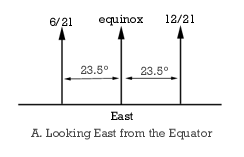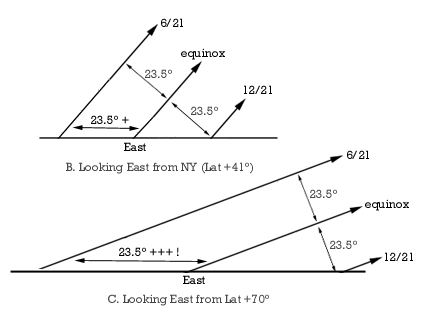Azimuth of SunriseFigure A: The paths of Solstice Suns are parallel to each other and are 23.5° N and S of the path of the Equinox Sun. At the Equator, where the Sun rises and sets perpendicular to the horizon, the Summer Solstice Sun rises 23.5° north of due East, and sets 23.5° north of due West.Figures B and C: At latitudes north and south of the Equator, the paths of the Solstice Suns are still 23.5° N and S of the path of the Equinox Sun, but measured along the horizon the angular separation is greater than 23.5°.
At latitudes near the equator, a reasonable approximation of the sunrise azimuth can be calculated using simple plane geometry. But since the horizon actually 'wraps around you', the approximation doesn't work as the angle between the horizon and the path of the Sun decreases (and the angular separation of the equinox and solstice sunrise increases) at higher latitudes.
The true angular separation of the solstice and equinox sunrise is calculated by the following:
sin(D) = sin(i)/ cos(l)
where D is the angular distance of sunrise and sunset from due east or west at the solstices, i is the tilt of Earth's axis and l is the observer's latitude.
Get an interactive spreadsheet and chart of sunrise azimuths.

Go HERE for more about sunrise and sunset times, length of daylight period, and the effects of atmospheric refraction.

Send comments to Steve Kluge at Resources for GeoScience Education